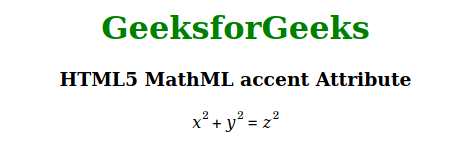# HTML5 MathML accent Attribute

• Last Updated : 18 Nov, 2020

The MathML accent attribute in HTML5 is used to specify whether an operator should be treated as an accent. The possible values of this attribute are true or false. This attribute can be used with <mo>, <mover> and <munderover> MathML tags.

Syntax:

```<element accent="true | false">
```

Attributes Value: This attribute has two values as mentioned above and described below:

• true: This value means the operator should be treated as an accent.
• false: This value means the operator should not be treated as an accent.

The example below illustrates the MathML accent attribute:

Example:

## HTML

 ```<``html``>`` ` `<``body` `style``=``"text-align:center;"``>`` ` `    ``<``h1` `style``=``"color:green"``>``        ``GeeksforGeeks``    ```` ` `    ``<``h3``>``        ``HTML5 MathML accent Attribute``    ```` ` `    ``<``math``>``        ``<``mrow``>``            ``<``mrow``>``                ``<``msup``>``                    ``<``mi``>x``                    ``<``mn``>2``                ````                ``<``mo` `accent``=``"true"``>+``                ``<``msup``>``                    ``<``mi``>y``                    ``<``mn``>2``                ````            ````            ``<``mo` `accent``=``"true"``>=``            ``<``msup``>``                ``<``mi``>z``                ``<``mn``>2``            ````        ````    `````` ` ``

Output:Supported Browsers: The browsers supported by HTML5 MathML accent attribute are listed below:

• Firefox

My Personal Notes arrow_drop_up